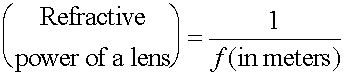Eye glasses and contact lenses The human eye has a lens that is able to form a real image on the back of the eye where receptors relay the signal to the brain. By flexing the lens the eye is able to focus objects located from a person's near point to their far point. The near point is the closest point at which a placed object can be brought into focus. A far point is the furthest point. A normal near point is 25 cm, while the normal far point is at infinity. If an individual's near point is outside the normal near point, eye glasses or contact lenses can be used to correct the matter. Such a person is far-sighted as the person can only focus objects far away. If an individual's far point is closer than infinity, that person is near-sighted. Corrective lenses can be either convex (converging) for far-sightedness or concave (diverging) for near-sightedness. Lenses with shorter focal lengths are stronger lenses, and the strength (refractive power) is measured in diopters, which is given by the inverse focal length, with the focal length measured in meters.The refractive power of a lens is positive for a convex (converging) lens and negative for a concave (diverging) lens. In the next two pages calculating a prescription for a lens will be demonstrated for near and far-sighted cases respectively.